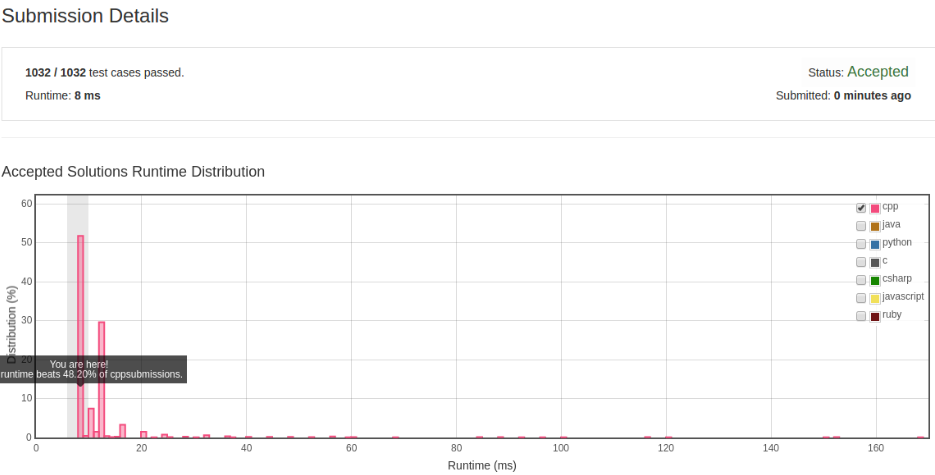# 凌云的博客

## LeetCode 算法题 7. Reverse Integer

### 题目

Reverse digits of an integer.

``````Example1: x = 123, return 321
Example2: x = -123, return -321
``````

Here are some good questions to ask before coding. Bonus points for you if you have already thought through this!

If the integer's last digit is 0, what should the output be? ie, cases such as 10, 100.

Did you notice that the reversed integer might overflow? Assume the input is a 32-bit integer, then the reverse of 1000000003 overflows. How should you handle such cases?

For the purpose of this problem, assume that your function returns 0 when the reversed integer overflows.

### 解法

``````int ret = 0;
while (x) {
ret = ret * 10 + x % 10;
x /= 10;
}
``````

``````class Solution {
public:
int reverse(int x) {
bool negative = false;
if (x < 0) {
negative = true;
x = -x;
}

long ret = 0;
while (x) {
ret = ret * 10 + (x % 10);
x /= 10;
}

if (negative) {
ret = - ret;
}

if (ret > INT_MAX || ret < INT_MIN) {
return 0;
}

return ret;
}
};
``````